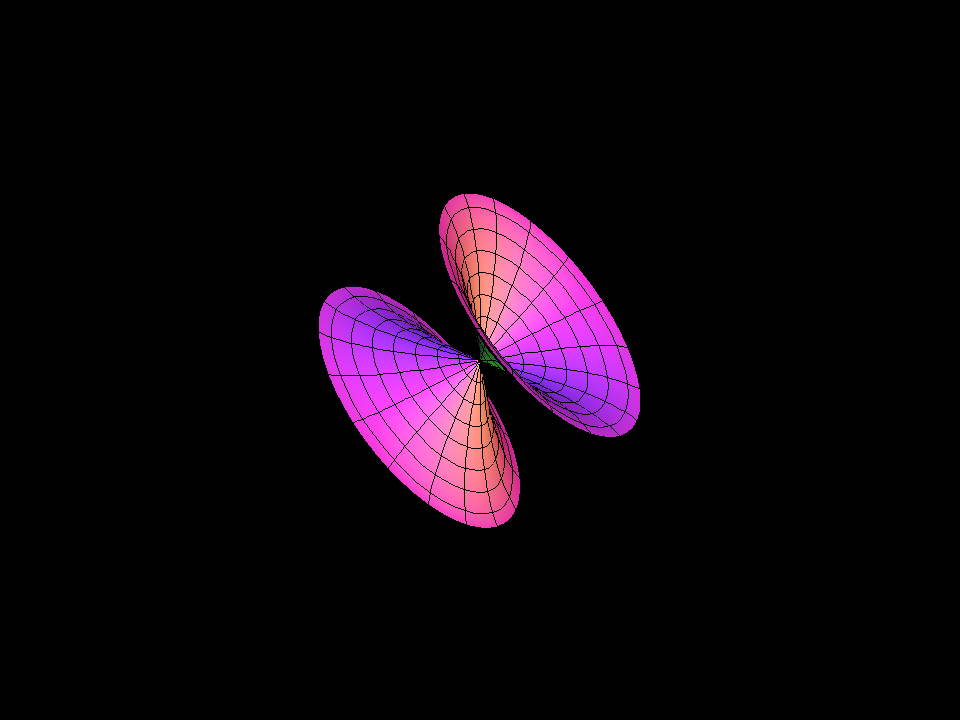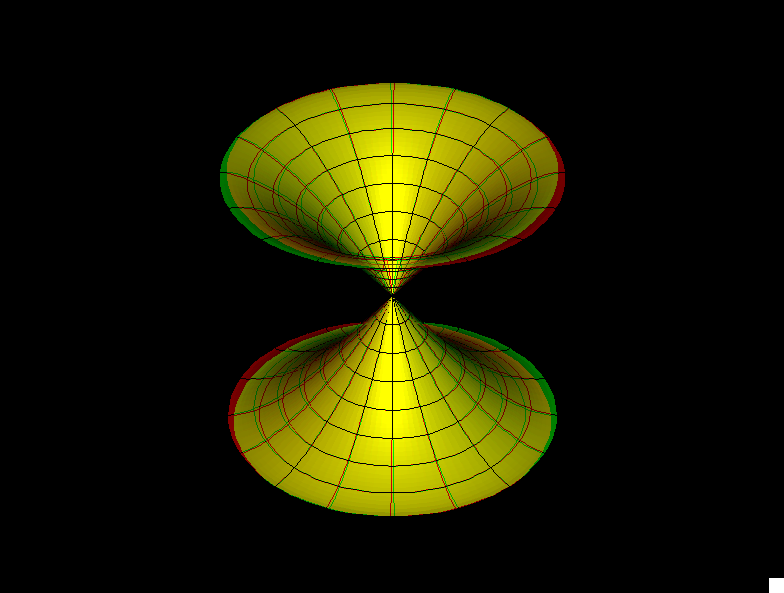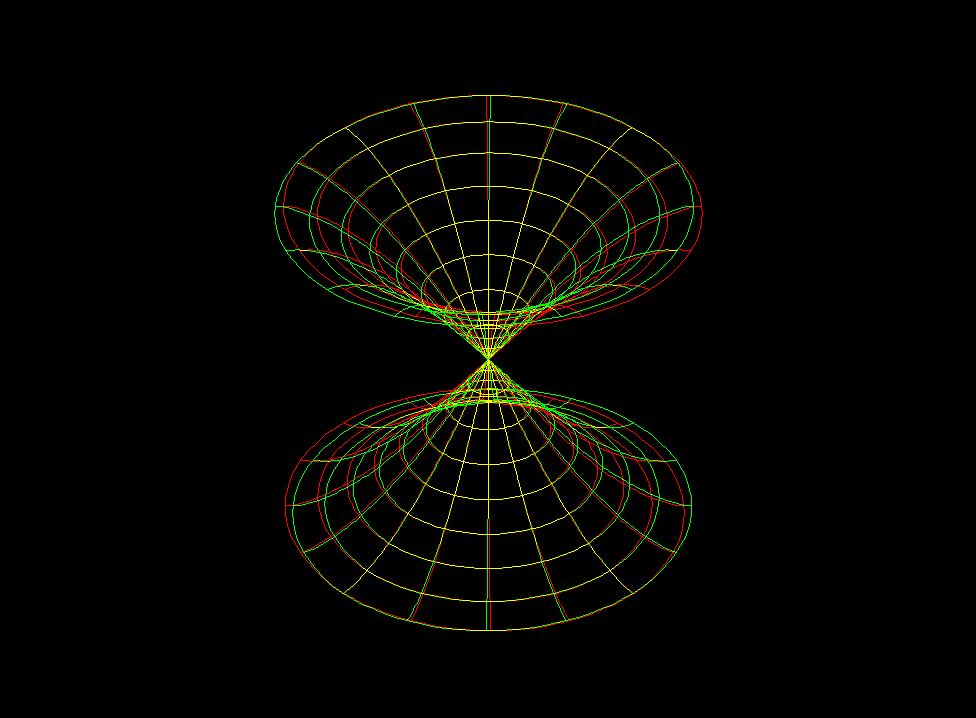# Conic K=-1 Surface of RevolutionConic K = -1 Surface of Revolution

One half of these surfaces (cut in the middle) converge to the Pseudosphere

The formulas for the meridians of the surfaces of revolution with Gauss curvature `K = -1` are explicit and well known. To deform these surfaces, keeping `K = -1`, it is more convenient to construct these surfaces from solutions of the Sine-Gordon equation (SGE). For surfaces of revolution these SGE solutions can be obtained from the ODE `q''(u) = sin(q(u))` , with skew-symmetric solutions defined by initial conditions

• `q(0) = 0`
• `q'(0) = b > 0`

Then one can do the same as in the classical Dini deformation: define new solutions

`qn(u,v) := q(cosh(d)*u + sinh(d)*v)`

, with d the Dini parameter. The animation shows these surfaces.An anaglyph wire frame sequence of the same deformation as before. The final surface has screw motion symmetry and no self intersections.conic k 1 sor stconic k 1 sor sw

Get red/blue stereo glasses from amazon

Conic_K=_-1_Surface_of_Revolution.pdf